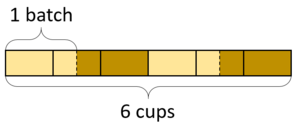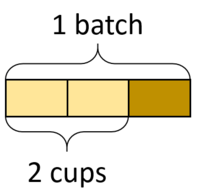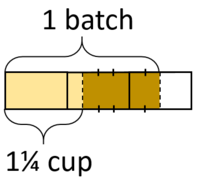# How Much in One Batch?

Alignments to Content Standards: 6.NS.A

10 cups of flour make 5 batches of cookies. How many cups of flour in one batch? Here are a diagram and a division equation that represent this situation:$$10\div5 = ?$$

For each question, draw a diagram and write a division equation.

1. 6 cups of flour make 4 batches of cupcakes. How many cups of flour in one batch?
2. 2 cups of flour make $\frac23$ of a batch of bread. How many cups of flour in one batch?
3. $1\frac{1}{4}$ cups of flour makes $\frac12$ a batch of rolls. How many cups of flour in one batch?

## IM Commentary

The purpose of this task is to help students extend their understanding of multiplication and division of whole numbers to multiplication and division of fractions. In grade 5, students

• multiply two fractions together and learn that a fraction times a number can be interpreted as that fraction of the number,
• divide two whole numbers with a non-whole number quotient, and
• divide a whole number by a unit fraction and a unit fraction by a whole number.

Notably, all of the division students do in grade 5 can be supported by an understanding of a whole number of groups. The big shift in grade 6 is that students tackle division contexts that involve a fractional number of groups.

The task does not ask students to find the quotient since the task is more about learning how to represent the situation, but teachers might choose to ask students to find or estimate the quotient, if desired.

This task helps students see how to represent How Much in a Group? contexts with division equations. It's designed to be used along with the task How Many Batches/What Fraction of a Batch? which covers the How Many Groups/What Fraction of a Group? situation.

To offload some of the tedium of drawing diagrams so that students can focus on thinking about sizes and numbers of batches, teachers might offer some printed templates for students to work on.

## Solution

1. 6 cups of flour make 4 batches of cupcakes. How many cups of flour in one batch?$$6 \div 4 = ?$$
2. 2 cups of flour make $\frac23$ of a batch of bread. How many cups of flour in one batch?$$2 \div \frac23 = ?$$
3. $1\frac{1}{4}$ cups of flour makes $\frac12$ a batch of rolls. How many cups of flour in one batch?$$1\frac14 \div \frac12 = ?$$